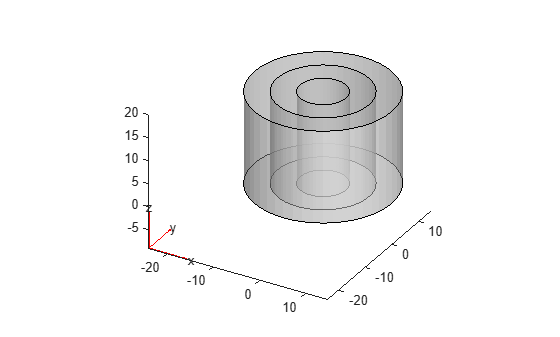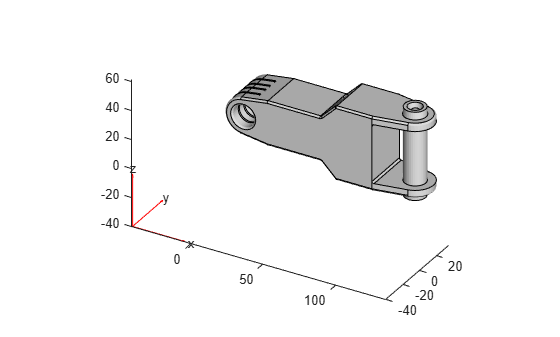# DiscreteGeometry

Discrete 2-D or 3-D geometry description

## Description

`DiscreteGeometry` describes a 2-D or 3-D geometry in the form of a discrete geometry object. `PDEModel`, `StructuralModel`, and `ThermalModel` objects have a `Geometry` property, which can be an `AnalyticGeometry` or `DiscreteGeometry` object.

## Creation

Create a discrete geometry for your model by using one of the following approaches:

## Properties

expand all

Number of geometry cells, specified as a nonnegative integer.

Data Types: `double`

Number of geometry edges, specified as a nonnegative integer.

Data Types: `double`

Number of geometry faces, specified as a positive integer.

Data Types: `double`

Number of geometry vertices, specified as a nonnegative integer.

Data Types: `double`

Coordinates of geometry vertices, specified as an `N`-by-2 or `N`-by-3 numeric matrix for a 2-D or 3-D geometry, respectively. Here, `N` is the number of vertices.

Data Types: `double`

## Object Functions

 `addFace` Fill void regions in 2-D and split cells in 3-D geometry `addVertex` Add vertex on geometry boundary `rotate` Rotate geometry `scale` Scale geometry `translate` Translate geometry `mergeCells` Merge geometry cells `cellEdges` Find edges belonging to boundaries of specified cells `cellFaces` Find faces belonging to specified cells `faceEdges` Find edges belonging to specified faces `facesAttachedToEdges` Find faces attached to specified edges `nearestEdge` Find edges nearest to specified point `nearestFace` Find faces nearest to specified point

## Examples

collapse all

Create the geometry consisting of three cylinders of the same height by using the `multicylinder` function.

`gm = multicylinder([5 10 15],20)`
```gm = DiscreteGeometry with properties: NumCells: 3 NumFaces: 9 NumEdges: 6 NumVertices: 6 Vertices: [6x3 double] ```

Plot the geometry.

`pdegplot(gm,FaceAlpha=0.5)`Import a geometry from an STL geometry file.

`gm = importGeometry("ForearmLink.stl")`
```gm = DiscreteGeometry with properties: NumCells: 1 NumFaces: 147 NumEdges: 329 NumVertices: 213 Vertices: [213x3 double] ```

Plot the geometry,

`pdegplot(gm)`## Version History

Introduced in R2015a